# Sample Papers for Class 11 Mathematics

• ### CBSE Class 11 Mathematics Sample Paper Set F

CBSE Class 11 Mathematics Sample Paper Set F. It’s always recommended to practice as many sample papers as possible before the examinations. Students can download the sample papers and also question papers of previous years to practice and score better marks in examinations. Refer to other links...

• ### CBSE Class 11 Mathematics Sample Paper Set G

CBSE Class 11 Mathematics Sample Paper Set G. It’s always recommended to practice as many sample papers as possible before the examinations. Students can download the sample papers and also question papers of previous years to practice and score better marks in examinations. Refer to other links...

• ### CBSE Class 11 Mathematics Sample Paper Set H

CBSE Class 11 Mathematics Sample Paper Set H. It’s always recommended to practice as many sample papers as possible before the examinations. Students can download the sample papers and also question papers of previous years to practice and score better marks in examinations. Refer to other links...

• ### CBSE Class 11 Mathematics Sample Paper Set I

CBSE Class 11 Mathematics Sample Paper Set I. It’s always recommended to practice as many sample papers as possible before the examinations. Students can download the sample papers and also question papers of previous years to practice and score better marks in examinations. Refer to other links...

• ### CBSE Class 11 Mathematics Sample Paper Set J

CBSE Class 11 Mathematics Sample Paper Set J. It’s always recommended to practice as many sample papers as possible before the examinations. Students can download the sample papers and also question papers of previous years to practice and score better marks in examinations. Refer to other links...

# CBSE Class 11 Mathematics Sample Paper Set F

CBSE Class 11 Mathematics Sample Paper Set F. It’s always recommended to practice as many sample papers as possible before the examinations. Students can download the sample papers and also question papers of previous years to practice and score better marks in examinations. Refer to other links too for more sample papers.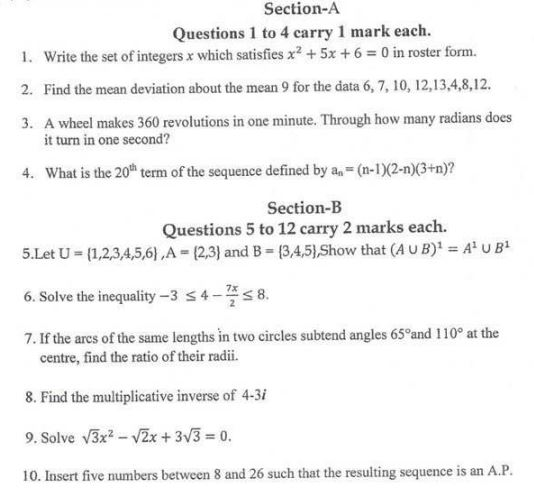Click for more Mathematics Study Material
 CBSE Class 11 Mathematics Sample Paper Set M CBSE Class 11 Mathematics Sample Paper Set H CBSE Class 11 Mathematics Sample Paper Set U CBSE Class 11 Mathematics Sample Paper Set D CBSE Class 11 Mathematics Sample Paper Set X CBSE Class 11 Mathematics Sample Paper Set Y CBSE Class 11 Mathematics Sample Paper Set G CBSE Class 11 Mathematics Sample Paper Set T CBSE Class 11 Mathematics Sample Paper Set C CBSE Class 11 Mathematics Sample Paper Set W CBSE Class 11 Mathematics Sample Paper Set S CBSE Class 11 Mathematics Sample Paper Set F CBSE Class 11 Mathematics Sample Paper Set Q CBSE Class 11 Mathematics Sample Paper Set B CBSE Class 11 Mathematics Sample Paper Set 3 CBSE Class 11 Mathematics Sample Paper Set R CBSE Class 11 Mathematics Sample Paper Set Z CBSE Class 11 Mathematics Sample Paper Set A CBSE Class 11 Mathematics Sample Paper Set 2 CBSE Class 11 Mathematics Sample Paper Set L CBSE Class 11 Mathematics Sample Paper Set 6 CBSE Class 11 Mathematics Sample Paper Set O CBSE Class 11 Mathematics Sample Paper Set J CBSE Class 11 Mathematics Sample Paper Set 1 CBSE Class 11 Mathematics Sample Paper Set K CBSE Class 11 Mathematics Sample Paper Set 5 CBSE Class 11 Mathematics Sample Paper Set N CBSE Class 11 Mathematics Sample Paper Set I CBSE Class 11 Mathematics Sample Paper Set V CBSE Class 11 Mathematics Sample Paper Set E CBSE Class 11 Mathematics Sample Paper Set 4 CBSE Class 11 Mathematics Sample Paper Set P

# CBSE Class 11 Mathematics Sample Paper Set G

CBSE Class 11 Mathematics Sample Paper Set G. It’s always recommended to practice as many sample papers as possible before the examinations. Students can download the sample papers and also question papers of previous years to practice and score better marks in examinations. Refer to other links too for more sample papers.Click for more Mathematics Study Material
 CBSE Class 11 Mathematics Sample Paper Set M CBSE Class 11 Mathematics Sample Paper Set H CBSE Class 11 Mathematics Sample Paper Set U CBSE Class 11 Mathematics Sample Paper Set D CBSE Class 11 Mathematics Sample Paper Set X CBSE Class 11 Mathematics Sample Paper Set Y CBSE Class 11 Mathematics Sample Paper Set G CBSE Class 11 Mathematics Sample Paper Set T CBSE Class 11 Mathematics Sample Paper Set C CBSE Class 11 Mathematics Sample Paper Set W CBSE Class 11 Mathematics Sample Paper Set S CBSE Class 11 Mathematics Sample Paper Set F CBSE Class 11 Mathematics Sample Paper Set Q CBSE Class 11 Mathematics Sample Paper Set B CBSE Class 11 Mathematics Sample Paper Set 3 CBSE Class 11 Mathematics Sample Paper Set R CBSE Class 11 Mathematics Sample Paper Set Z CBSE Class 11 Mathematics Sample Paper Set A CBSE Class 11 Mathematics Sample Paper Set 2 CBSE Class 11 Mathematics Sample Paper Set L CBSE Class 11 Mathematics Sample Paper Set 6 CBSE Class 11 Mathematics Sample Paper Set O CBSE Class 11 Mathematics Sample Paper Set J CBSE Class 11 Mathematics Sample Paper Set 1 CBSE Class 11 Mathematics Sample Paper Set K CBSE Class 11 Mathematics Sample Paper Set 5 CBSE Class 11 Mathematics Sample Paper Set N CBSE Class 11 Mathematics Sample Paper Set I CBSE Class 11 Mathematics Sample Paper Set V CBSE Class 11 Mathematics Sample Paper Set E CBSE Class 11 Mathematics Sample Paper Set 4 CBSE Class 11 Mathematics Sample Paper Set P

# CBSE Class 11 Mathematics Sample Paper Set H

CBSE Class 11 Mathematics Sample Paper Set H. It’s always recommended to practice as many sample papers as possible before the examinations. Students can download the sample papers and also question papers of previous years to practice and score better marks in examinations. Refer to other links too for more sample papers.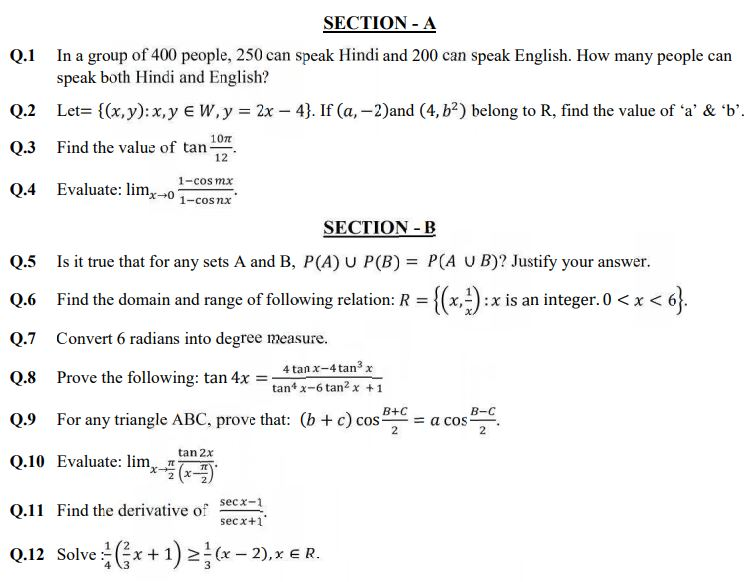Click for more Mathematics Study Material
 CBSE Class 11 Mathematics Sample Paper Set M CBSE Class 11 Mathematics Sample Paper Set H CBSE Class 11 Mathematics Sample Paper Set U CBSE Class 11 Mathematics Sample Paper Set D CBSE Class 11 Mathematics Sample Paper Set X CBSE Class 11 Mathematics Sample Paper Set Y CBSE Class 11 Mathematics Sample Paper Set G CBSE Class 11 Mathematics Sample Paper Set T CBSE Class 11 Mathematics Sample Paper Set C CBSE Class 11 Mathematics Sample Paper Set W CBSE Class 11 Mathematics Sample Paper Set S CBSE Class 11 Mathematics Sample Paper Set F CBSE Class 11 Mathematics Sample Paper Set Q CBSE Class 11 Mathematics Sample Paper Set B CBSE Class 11 Mathematics Sample Paper Set 3 CBSE Class 11 Mathematics Sample Paper Set R CBSE Class 11 Mathematics Sample Paper Set Z CBSE Class 11 Mathematics Sample Paper Set A CBSE Class 11 Mathematics Sample Paper Set 2 CBSE Class 11 Mathematics Sample Paper Set L CBSE Class 11 Mathematics Sample Paper Set 6 CBSE Class 11 Mathematics Sample Paper Set O CBSE Class 11 Mathematics Sample Paper Set J CBSE Class 11 Mathematics Sample Paper Set 1 CBSE Class 11 Mathematics Sample Paper Set K CBSE Class 11 Mathematics Sample Paper Set 5 CBSE Class 11 Mathematics Sample Paper Set N CBSE Class 11 Mathematics Sample Paper Set I CBSE Class 11 Mathematics Sample Paper Set V CBSE Class 11 Mathematics Sample Paper Set E CBSE Class 11 Mathematics Sample Paper Set 4 CBSE Class 11 Mathematics Sample Paper Set P

# CBSE Class 11 Mathematics Sample Paper Set I

CBSE Class 11 Mathematics Sample Paper Set I. It’s always recommended to practice as many sample papers as possible before the examinations. Students can download the sample papers and also question papers of previous years to practice and score better marks in examinations. Refer to other links too for more sample papers.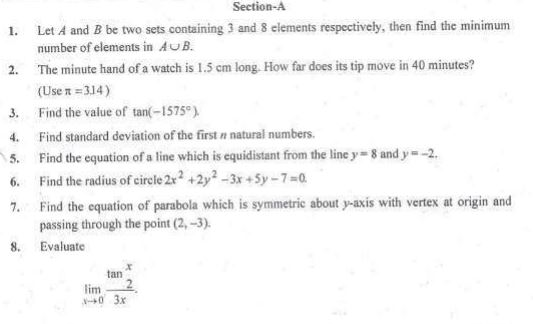Click for more Mathematics Study Material
 CBSE Class 11 Mathematics Sample Paper Set M CBSE Class 11 Mathematics Sample Paper Set H CBSE Class 11 Mathematics Sample Paper Set U CBSE Class 11 Mathematics Sample Paper Set D CBSE Class 11 Mathematics Sample Paper Set X CBSE Class 11 Mathematics Sample Paper Set Y CBSE Class 11 Mathematics Sample Paper Set G CBSE Class 11 Mathematics Sample Paper Set T CBSE Class 11 Mathematics Sample Paper Set C CBSE Class 11 Mathematics Sample Paper Set W CBSE Class 11 Mathematics Sample Paper Set S CBSE Class 11 Mathematics Sample Paper Set F CBSE Class 11 Mathematics Sample Paper Set Q CBSE Class 11 Mathematics Sample Paper Set B CBSE Class 11 Mathematics Sample Paper Set 3 CBSE Class 11 Mathematics Sample Paper Set R CBSE Class 11 Mathematics Sample Paper Set Z CBSE Class 11 Mathematics Sample Paper Set A CBSE Class 11 Mathematics Sample Paper Set 2 CBSE Class 11 Mathematics Sample Paper Set L CBSE Class 11 Mathematics Sample Paper Set 6 CBSE Class 11 Mathematics Sample Paper Set O CBSE Class 11 Mathematics Sample Paper Set J CBSE Class 11 Mathematics Sample Paper Set 1 CBSE Class 11 Mathematics Sample Paper Set K CBSE Class 11 Mathematics Sample Paper Set 5 CBSE Class 11 Mathematics Sample Paper Set N CBSE Class 11 Mathematics Sample Paper Set I CBSE Class 11 Mathematics Sample Paper Set V CBSE Class 11 Mathematics Sample Paper Set E CBSE Class 11 Mathematics Sample Paper Set 4 CBSE Class 11 Mathematics Sample Paper Set P

# CBSE Class 11 Mathematics Sample Paper Set J

CBSE Class 11 Mathematics Sample Paper Set J. It’s always recommended to practice as many sample papers as possible before the examinations. Students can download the sample papers and also question papers of previous years to practice and score better marks in examinations. Refer to other links too for more sample papers.Click for more Mathematics Study Material
 CBSE Class 11 Mathematics Sample Paper Set M CBSE Class 11 Mathematics Sample Paper Set H CBSE Class 11 Mathematics Sample Paper Set U CBSE Class 11 Mathematics Sample Paper Set D CBSE Class 11 Mathematics Sample Paper Set X CBSE Class 11 Mathematics Sample Paper Set Y CBSE Class 11 Mathematics Sample Paper Set G CBSE Class 11 Mathematics Sample Paper Set T CBSE Class 11 Mathematics Sample Paper Set C CBSE Class 11 Mathematics Sample Paper Set W CBSE Class 11 Mathematics Sample Paper Set S CBSE Class 11 Mathematics Sample Paper Set F CBSE Class 11 Mathematics Sample Paper Set Q CBSE Class 11 Mathematics Sample Paper Set B CBSE Class 11 Mathematics Sample Paper Set 3 CBSE Class 11 Mathematics Sample Paper Set R CBSE Class 11 Mathematics Sample Paper Set Z CBSE Class 11 Mathematics Sample Paper Set A CBSE Class 11 Mathematics Sample Paper Set 2 CBSE Class 11 Mathematics Sample Paper Set L CBSE Class 11 Mathematics Sample Paper Set 6 CBSE Class 11 Mathematics Sample Paper Set O CBSE Class 11 Mathematics Sample Paper Set J CBSE Class 11 Mathematics Sample Paper Set 1 CBSE Class 11 Mathematics Sample Paper Set K CBSE Class 11 Mathematics Sample Paper Set 5 CBSE Class 11 Mathematics Sample Paper Set N CBSE Class 11 Mathematics Sample Paper Set I CBSE Class 11 Mathematics Sample Paper Set V CBSE Class 11 Mathematics Sample Paper Set E CBSE Class 11 Mathematics Sample Paper Set 4 CBSE Class 11 Mathematics Sample Paper Set P

# CBSE Class 11 Mathematics Sample Paper Set A

CBSE Class 11 Mathematics Sample Paper Set A. It’s always recommended to practice as many sample papers as possible before the examinations. Students can download the sample papers and also question papers of previous years to practice and score better marks in examinations. Refer to other links too for more sample papers.

SECTION-A

Q1. Write the contrapositive of the following statement. If a triangle is equilateral, it is isosceles.

Click for more Mathematics Study Material
 CBSE Class 11 Mathematics Sample Paper Set M CBSE Class 11 Mathematics Sample Paper Set H CBSE Class 11 Mathematics Sample Paper Set U CBSE Class 11 Mathematics Sample Paper Set D CBSE Class 11 Mathematics Sample Paper Set X CBSE Class 11 Mathematics Sample Paper Set Y CBSE Class 11 Mathematics Sample Paper Set G CBSE Class 11 Mathematics Sample Paper Set T CBSE Class 11 Mathematics Sample Paper Set C CBSE Class 11 Mathematics Sample Paper Set W CBSE Class 11 Mathematics Sample Paper Set S CBSE Class 11 Mathematics Sample Paper Set F CBSE Class 11 Mathematics Sample Paper Set Q CBSE Class 11 Mathematics Sample Paper Set B CBSE Class 11 Mathematics Sample Paper Set 3 CBSE Class 11 Mathematics Sample Paper Set R CBSE Class 11 Mathematics Sample Paper Set Z CBSE Class 11 Mathematics Sample Paper Set A CBSE Class 11 Mathematics Sample Paper Set 2 CBSE Class 11 Mathematics Sample Paper Set L CBSE Class 11 Mathematics Sample Paper Set 6 CBSE Class 11 Mathematics Sample Paper Set O CBSE Class 11 Mathematics Sample Paper Set J CBSE Class 11 Mathematics Sample Paper Set 1 CBSE Class 11 Mathematics Sample Paper Set K CBSE Class 11 Mathematics Sample Paper Set 5 CBSE Class 11 Mathematics Sample Paper Set N CBSE Class 11 Mathematics Sample Paper Set I CBSE Class 11 Mathematics Sample Paper Set V CBSE Class 11 Mathematics Sample Paper Set E CBSE Class 11 Mathematics Sample Paper Set 4 CBSE Class 11 Mathematics Sample Paper Set P

# CBSE Class 11 Mathematics Sample Paper Set B

CBSE Class 11 Mathematics Sample Paper Set B. It’s always recommended to practice as many sample papers as possible before the examinations. Students can download the sample papers and also question papers of previous years to practice and score better marks in examinations. Refer to other links too for more sample papers.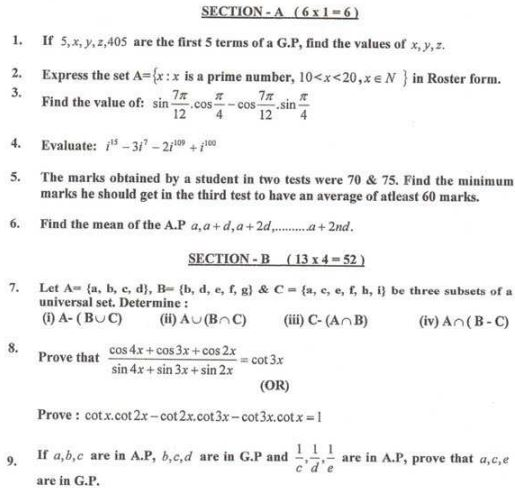Click for more Mathematics Study Material
 CBSE Class 11 Mathematics Sample Paper Set M CBSE Class 11 Mathematics Sample Paper Set H CBSE Class 11 Mathematics Sample Paper Set U CBSE Class 11 Mathematics Sample Paper Set D CBSE Class 11 Mathematics Sample Paper Set X CBSE Class 11 Mathematics Sample Paper Set Y CBSE Class 11 Mathematics Sample Paper Set G CBSE Class 11 Mathematics Sample Paper Set T CBSE Class 11 Mathematics Sample Paper Set C CBSE Class 11 Mathematics Sample Paper Set W CBSE Class 11 Mathematics Sample Paper Set S CBSE Class 11 Mathematics Sample Paper Set F CBSE Class 11 Mathematics Sample Paper Set Q CBSE Class 11 Mathematics Sample Paper Set B CBSE Class 11 Mathematics Sample Paper Set 3 CBSE Class 11 Mathematics Sample Paper Set R CBSE Class 11 Mathematics Sample Paper Set Z CBSE Class 11 Mathematics Sample Paper Set A CBSE Class 11 Mathematics Sample Paper Set 2 CBSE Class 11 Mathematics Sample Paper Set L CBSE Class 11 Mathematics Sample Paper Set 6 CBSE Class 11 Mathematics Sample Paper Set O CBSE Class 11 Mathematics Sample Paper Set J CBSE Class 11 Mathematics Sample Paper Set 1 CBSE Class 11 Mathematics Sample Paper Set K CBSE Class 11 Mathematics Sample Paper Set 5 CBSE Class 11 Mathematics Sample Paper Set N CBSE Class 11 Mathematics Sample Paper Set I CBSE Class 11 Mathematics Sample Paper Set V CBSE Class 11 Mathematics Sample Paper Set E CBSE Class 11 Mathematics Sample Paper Set 4 CBSE Class 11 Mathematics Sample Paper Set P

# CBSE Class 11 Mathematics Sample Paper Set C

CBSE Class 11 Mathematics Sample Paper Set C. It’s always recommended to practice as many sample papers as possible before the examinations. Students can download the sample papers and also question papers of previous years to practice and score better marks in examinations. Refer to other links too for more sample papers.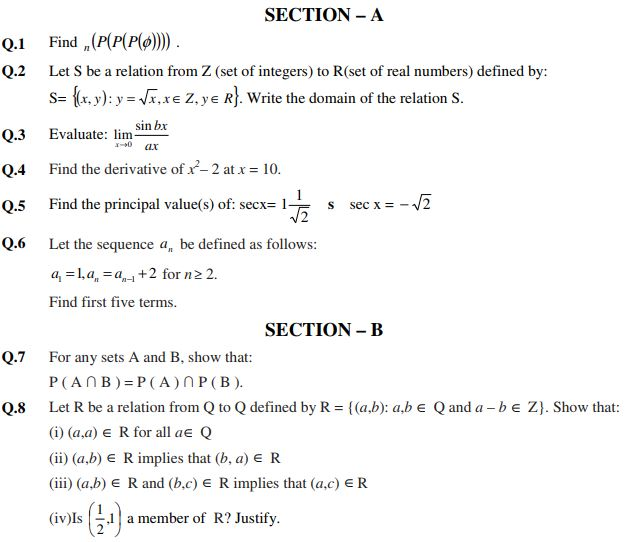Click for more Mathematics Study Material
 CBSE Class 11 Mathematics Sample Paper Set M CBSE Class 11 Mathematics Sample Paper Set H CBSE Class 11 Mathematics Sample Paper Set U CBSE Class 11 Mathematics Sample Paper Set D CBSE Class 11 Mathematics Sample Paper Set X CBSE Class 11 Mathematics Sample Paper Set Y CBSE Class 11 Mathematics Sample Paper Set G CBSE Class 11 Mathematics Sample Paper Set T CBSE Class 11 Mathematics Sample Paper Set C CBSE Class 11 Mathematics Sample Paper Set W CBSE Class 11 Mathematics Sample Paper Set S CBSE Class 11 Mathematics Sample Paper Set F CBSE Class 11 Mathematics Sample Paper Set Q CBSE Class 11 Mathematics Sample Paper Set B CBSE Class 11 Mathematics Sample Paper Set 3 CBSE Class 11 Mathematics Sample Paper Set R CBSE Class 11 Mathematics Sample Paper Set Z CBSE Class 11 Mathematics Sample Paper Set A CBSE Class 11 Mathematics Sample Paper Set 2 CBSE Class 11 Mathematics Sample Paper Set L CBSE Class 11 Mathematics Sample Paper Set 6 CBSE Class 11 Mathematics Sample Paper Set O CBSE Class 11 Mathematics Sample Paper Set J CBSE Class 11 Mathematics Sample Paper Set 1 CBSE Class 11 Mathematics Sample Paper Set K CBSE Class 11 Mathematics Sample Paper Set 5 CBSE Class 11 Mathematics Sample Paper Set N CBSE Class 11 Mathematics Sample Paper Set I CBSE Class 11 Mathematics Sample Paper Set V CBSE Class 11 Mathematics Sample Paper Set E CBSE Class 11 Mathematics Sample Paper Set 4 CBSE Class 11 Mathematics Sample Paper Set P

# CBSE Class 11 Mathematics Sample Paper Set D

CBSE Class 11 Mathematics Sample Paper Set D. It’s always recommended to practice as many sample papers as possible before the examinations. Students can download the sample papers and also question papers of previous years to practice and score better marks in examinations. Refer to other links too for more sample papers.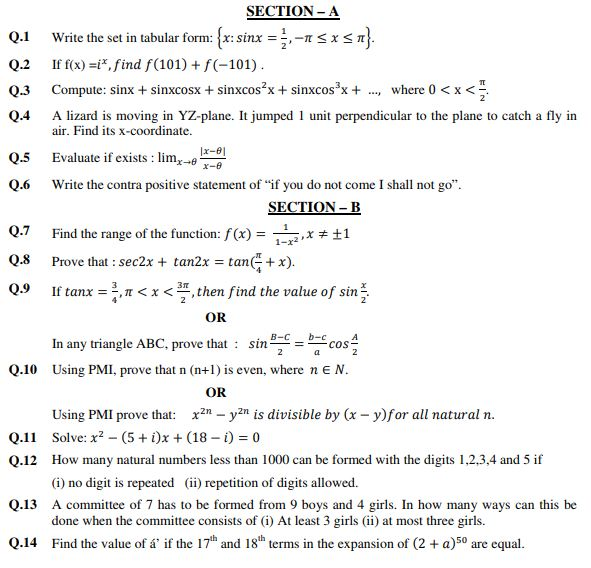Click for more Mathematics Study Material
 CBSE Class 11 Mathematics Sample Paper Set M CBSE Class 11 Mathematics Sample Paper Set H CBSE Class 11 Mathematics Sample Paper Set U CBSE Class 11 Mathematics Sample Paper Set D CBSE Class 11 Mathematics Sample Paper Set X CBSE Class 11 Mathematics Sample Paper Set Y CBSE Class 11 Mathematics Sample Paper Set G CBSE Class 11 Mathematics Sample Paper Set T CBSE Class 11 Mathematics Sample Paper Set C CBSE Class 11 Mathematics Sample Paper Set W CBSE Class 11 Mathematics Sample Paper Set S CBSE Class 11 Mathematics Sample Paper Set F CBSE Class 11 Mathematics Sample Paper Set Q CBSE Class 11 Mathematics Sample Paper Set B CBSE Class 11 Mathematics Sample Paper Set 3 CBSE Class 11 Mathematics Sample Paper Set R CBSE Class 11 Mathematics Sample Paper Set Z CBSE Class 11 Mathematics Sample Paper Set A CBSE Class 11 Mathematics Sample Paper Set 2 CBSE Class 11 Mathematics Sample Paper Set L CBSE Class 11 Mathematics Sample Paper Set 6 CBSE Class 11 Mathematics Sample Paper Set O CBSE Class 11 Mathematics Sample Paper Set J CBSE Class 11 Mathematics Sample Paper Set 1 CBSE Class 11 Mathematics Sample Paper Set K CBSE Class 11 Mathematics Sample Paper Set 5 CBSE Class 11 Mathematics Sample Paper Set N CBSE Class 11 Mathematics Sample Paper Set I CBSE Class 11 Mathematics Sample Paper Set V CBSE Class 11 Mathematics Sample Paper Set E CBSE Class 11 Mathematics Sample Paper Set 4 CBSE Class 11 Mathematics Sample Paper Set P

## Latest NCERT & CBSE News

Read the latest news and announcements from NCERT and CBSE below. Important updates relating to your studies which will help you to keep yourself updated with latest happenings in school level education. Keep yourself updated with all latest news and also read articles from teachers which will help you to improve your studies, increase motivation level and promote faster learning

### Score well in Class 12 English Boards Exam

12th Board exams are an important part of students' lives. The marks obtained in the board exam decide the college in which one can study. In class 12 the syllabus of each and every subject increases vastly and it is difficult to cover up every point. In English also...

### How To Solve Unseen Passages In English

Unseen passages may contain one or many paragraphs. This is one of the important yet easy parts for a student to get marks. Students should thoroughly study and understand the passage to answer the related questions. The unseen passages are there just to test the...

### Moderation of Marks Class 11 and 12 Board Exams

The portal for moderation and finalization of results for Class-12 is being opened from 16.07.2021 to 22.07.2021. As Board has to declare the result latest by 31.07.2021, schools have been requested to follow the schedule strictly and complete the moderation within...

### MCQ Question based CBSE examination

For 2021-22 CBSE has launched MCQ question-based examination for Term 1 & Term 2 board examinations. The entire syllabus has been divided into two parts each including 50% of the entire syllabus. To score well, students must practice as per the new CBSE term-wise...

### CBSE Class 10 Revised Syllabus

Last year CBSE had to reduce the syllabus because of the pandemic situation but it was not very effective because there were no examinations. This year to avoid any confusion and conflict, CBSE has decided to reduce the syllabus into term 1 and term 2. 50 percent of...

×